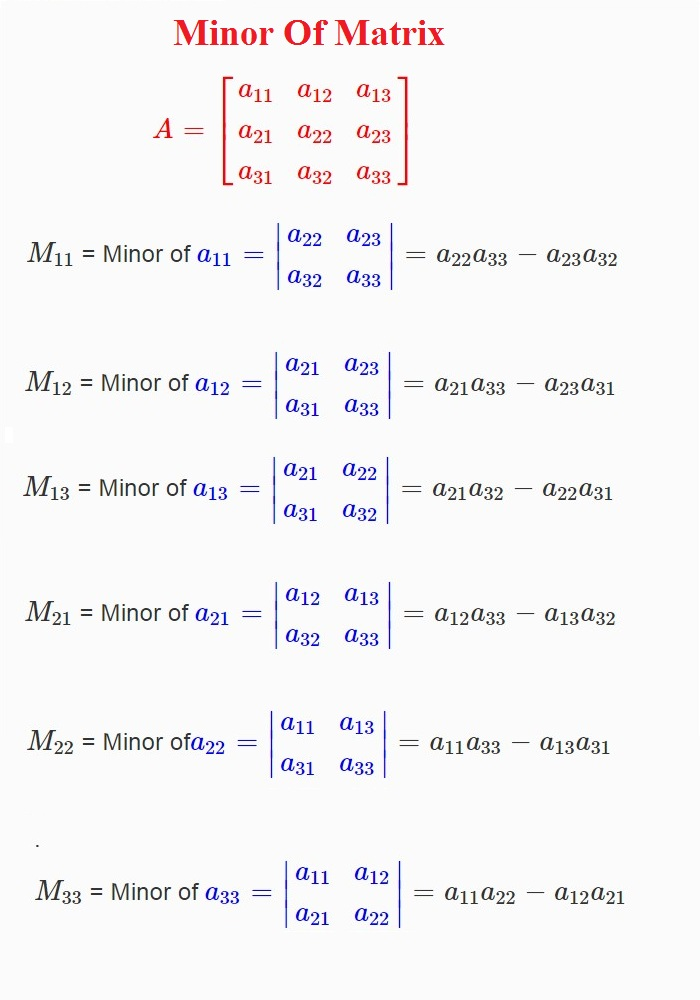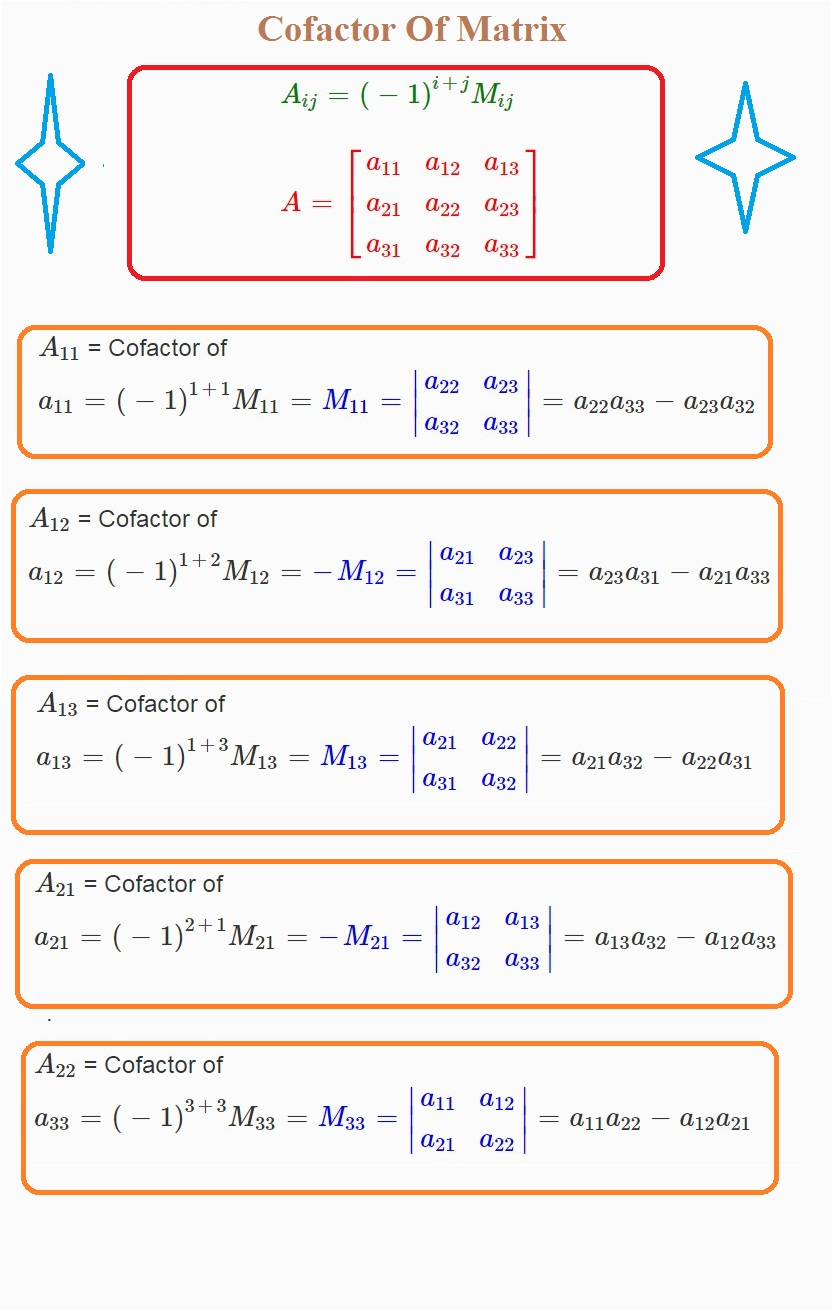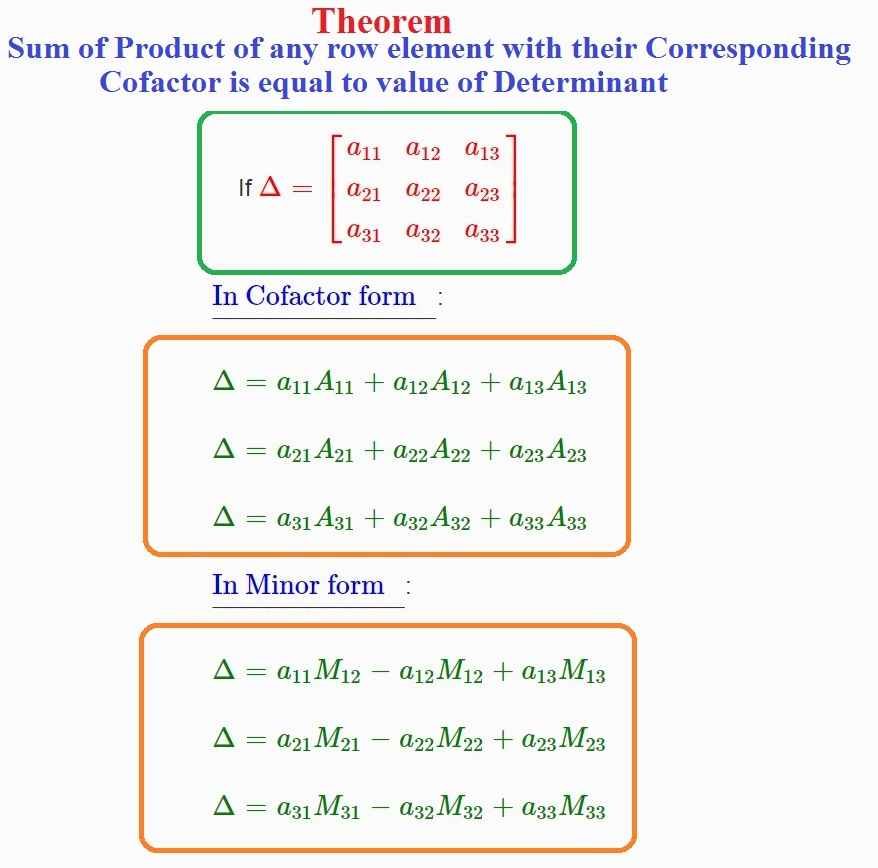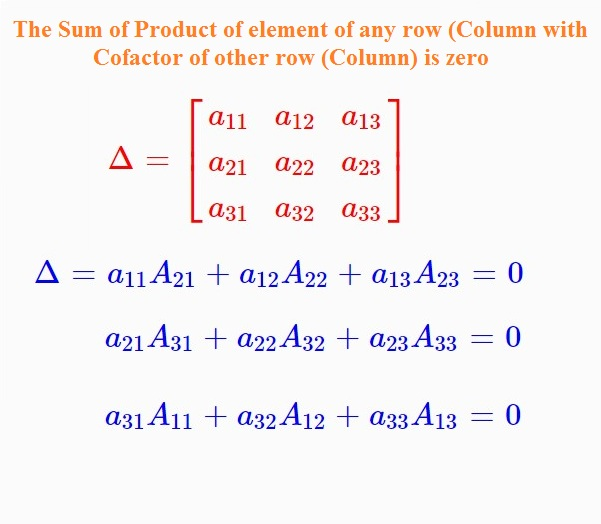Mathematics Area of a Triangle, Minors and Cofactors For CBSE-NCERT
Click for Only Video

### Topic covered

star Area of a Triangle
starMinors and Cofactors

### Area of a Triangle

color{green} ✍️ The area of triangle whose vertices are (x_1,y_1) , (x_2,y_2) and (x_3,y_3)

= |1/2[x_1(y_2 -y_3) + x_2(y_3-y_1) + x_3(y_1-y_2)]|

● Now this expression can be written in the form of a determinant as

color{red}{"Area =" 1/2 |(x_1,y_1,1),(x_2,y_2,1),(x_3,y_3,1) |}

Note : 1 . Area is a positive quantity, we always take the absolute value of the determinant.
2.color{orange}{"The area of the triangle formed by three collinear points is zero"}
3. If area is given, use both positive and negative values of the determinant for calculation.
Q 3124591451Find the area of the triangle whose vertices are (3, 8), (– 4, 2) and (5, 1).
Class 12 Chapter 4 Example 17Solution:

The area of triangle is given by

 Δ =1/2 | ( 3,8,1), (-4,2,1), (5,1,1) |

= 1/2 [ 3 (2-1) -8 (-4-5) +1 (-4 -10 ) ]

= 1/2 (3+72 -14) = 61/2
Q 3144591453Find the equation of the line joining A(1, 3) and B (0, 0) using determinants
and find k if D(k, 0) is a point such that area of triangle ABD is 3sq units.
Class 12 Chapter 4 Example 18Solution:

Let P (x, y) be any point on AB. Then, area of triangle ABP is zero (Why?). So

 1/2 | ( 0,0,1), ( 1,3,1) , (x,y,1) | =0

This gives  1/2 (y-3x) = 0 or y = 3x,

which is the equation of required line AB.
Also, since the area of the triangle ABD is 3 sq. units, we have

 1/2 | (1,3,1), (0,0,1), (k,0,1) | = pm 3

This gives,  (-3k)/2 = pm 3 i.e.,  k = ∓ 2.

### Minors Of Matrixcolor{green} ✍️ Minor of an element a_(ij) of a determinant is the determinant obtained by deleting its i^(th) row and j^(th) column in which element a_(ij) lies. Minor of an element a_(ij) is denoted by M_(ij) .

color {brown} text{Minor of Matrix }

color{red} {A = [ (a_11, a_12 , a_13 ), (a_21, a_22 , a_23 ),( a_31 ,a_32 ,a_33 )] }

M_11 = Minor of color {blue} {a_11 = | (a_22, a_23), ( a_32 , a_33 ) |} = a_22 a_33 - a_23 a_32

M_12 = Minor of color {blue} {a_12 = | ( a_21 , a_23 ), ( a_31 ,a_33 ) | } = a_21a_33 - a_23 a_31

M_13 = Minor of color {blue} {a_13 = | ( a_21 , a_22 ), ( a_31 ,a_32 ) | }= a_21a_32 - a_22 a_31

M_21 = Minor of color {blue} {a_21 = | ( a_12 , a_13 ), ( a_32 ,a_33 ) | } = a_12a_33 - a_13 a_32

M_22 = Minor ofcolor {blue} {a_22 = | ( a_11 , a_13 ), ( a_31 ,a_33 ) | } = a_11a_33 - a_13 a_31
.
.
.
.
.
M_33 = Minor of color {blue} {a_33 = | ( a_11 , a_12 ), ( a_21 ,a_22 ) | }= a_11a_22 - a_12 a_21

Remark : Minor of an element of a determinant of order n(n ≥ 2) is a determinant of order n – 1.
Q 3104591458Find the minor of element 6 in the determinant  Δ = | (1,2,3), (4,5,6), ( 7,8,9) |

Class 12 Chapter 4 Example 19Solution:

Since 6 lies in the second row and third column, its minor M_(23) is given by

M_(23) = | (1,2),(7,8) | = 8 -14 = - 6 (obtained by deleting R_2 and C_3 in Δ).

### Cofactor of Matrixcolor{green} ✍️ Cofactor of an element a_(ij) , denoted by A_(ij) is defined by

=>color{red} {"cofactor of element " a_(ij) "is define as " A_(ij) = (-1)^(i+j)M_(ij)}

color{red} {A = [ (a_11, a_12 , a_13 ), (a_21, a_22 , a_23 ),( a_31 ,a_32 ,a_33 )] }

A_11 = Cofactor of a_11 = (-1)^(1 +1)M_11 = color {blue} {M_11 = | (a_22, a_23), ( a_32 , a_33 ) |} = a_22 a_33 - a_23 a_32

A_12 = Cofactor of a_12 = (-1)^(1 +2)M_12 = color {blue} { - M_12 = | ( a_21 , a_23 ), ( a_31 ,a_33 ) | } = a_23 a_31 - a_21a_33

A_13 = Cofactor of a_13 = (-1)^(1 +3)M_13 = color {blue} {M_13 = | ( a_21 , a_22 ), ( a_31 ,a_32 ) | }= a_21a_32 - a_22 a_31

A_21 = Cofactor of a_21 = (-1)^(2 +1)M_21 = color {blue} { - M_21 = | ( a_12 , a_13 ), ( a_32 ,a_33 ) | } =a_13 a_32 - a_12a_33
.
.
.
.
.
.

A_22 = Cofactor of a_33 = (-1)^(3 +3)M_33 = color {blue} {M_33 = | ( a_11 , a_12 ), ( a_21 ,a_22 ) | }= a_11a_22 - a_12 a_21
Q 3114691550Find minors and cofactors of all the elements of the determinant | (1,-2), (4,3) |
Class 12 Chapter 4 Example 20Solution:

Minor of the element a_(ij) is M_(ij)

Here a_11 = 1. So M_11 = Minor of a_11= 3

M_12 = Minor of the element a_12 = 4

M_21 = Minor of the element a_21 = –2

M_22 = Minor of the element a_22 = 1

Now, cofactor of a_(ij) is A_ij. So

A_(11) = (–1)^(1 + 1) M_(11 ) = (–1)^2 (3) = 3

A_(12) = (–1)^(1 + 2) M_(12) = (–1)^3 (4) = – 4

A_(21 ) = (–1)^(2 + 1) M_(21) = (–1)^3 (–2) = 2

A_(22) = (–1)^(2 + 2) M_(22) = (–1)^4 (1) = 1
Q 3134691552Find minors and cofactors of the elements a_11, a_21  in the determinant

 Δ = | (a_11 ,a_12 , a_13), ( a_21 , a_22, a_23), ( a_31, a_32 , a_33) |
Class 12 Chapter 4 Example 21Solution:

By definition of minors and cofactors, we have

Minor of a_11 = M_11 = | (a_22, a_23), ( a_32 , a_33) | = a_22 a_33– a_23 a_32

Cofactor of a_11 = A_11 = (-1)^(1+1) M_11 = a_22 a_33 – a_23 a_32

Minor of a_21 = M_21 = | (a_12 , a_13), ( a_32 , a_33) | = a_12 a_33 – a_13 a_32

Cofactor of a_21 = A_21 = (–1)^(2+1) M_21 = (–1) (a_12 a_33 – a_13 a_32) = – a_12 a_33 + a_13 a_32
Q 3165101965Find minors and cofactors of the elements of the determinant

| (2,-3,5), (6,0,4), (1,5,-7) | and verify that a_11 A_31 + a_12 A_32 + a_13 A_33= 0
Class 12 Chapter 4 Example 22Solution:

We have M_11 = | ( 0,4), ( 5,-7) | = 0 –20 = –20; A_11 = (–1)^(1+1) (–20) = –20

M_12 = | (6,4), ( 1,-7) | = -42 -4 = -46 ; A_12 = (-1)^(1+2) (-46) = 46

M_13 = | (6,0 ), (1,5) | = 30 - 0 = 30; A_13 = (-1)^(1+3) (30) = 30

M_21 = | ( -3,5), (5,-7) | = 21 – 25 = – 4; A_21 = (–1)^(2+1 ) (– 4) = 4

M_22 = | (2,5), (1,-7) | = –14 – 5 = –19; A_22 = (–1)^(2+2) (–19) = –19

M_23 = | (2,-3), ( 1,5) | = 10 + 3 = 13; A_23 = (–1)^(2+3) (13) = –13

M_31 = | (-3,5), ( 0,4) | = –12 – 0 = –12; A_31 = (–1)3+1 (–12) = –12

M_32 = | (2,5), (6,4) | = 8 - 30 = -22 ; A_32 = (-1)^(3+2) (-22) = 22

and M_33 = | (2,-3), ( 6,0) | = 0 + 18 = 18; A_33 = (–1)^(3+3) (18) = 18

Now a_11 = 2, a_12 = –3, a_13 = 5; A_31 = –12, A_32 = 22, A_33 = 18

So a_11 A_31 + a_12 A_32 + a_13 A_33

= 2 (–12) + (–3) (22) + 5 (18) = –24 – 66 + 90 = 0

### Theorem : Sum of Product of any element with their corresponding Cofactor is equal to the value of Determinants.● Expanding the determinant Δ, along R_1, we have

 Δ = (-1)^(1+1) a_11 | (a_22, a_23), ( a_32 , a_33) | + (-1)^(1+2) a_12 | (a_21 , a_23), ( a_31, a_33) | + (-1)^(1+3) a_13 | ( a_21 , a_22 ), ( a_31 , a_32) |

= a_11 A_11 + a_12 A_12 + a_13 A_13, where A_(ij) is cofactor of a_(ij)

color{orange}{Δ=" sum of product of elements of " R_1" with their corresponding cofactors"}

●Hence, Sum of Product of any element with their corresponding Cofactor is equal to the value of Determinants.

ul color {blue } {text (In Cofactor form ) }:

color {green} {Delta = a_11 A_11 + a_12 A_12 + a_13 A_13}

color {green} { Delta = a_21 A_21 + a_22 A_22 + a_23 A_23}

color {green} { Delta = a_31 A_31 + a_32 A_32 + a_33 A_33}

ul color {blue } {text (In Minor form ) }:

color{green} {Delta = a_11 M_12 - a_12 M_12 + a_13 M_13}

color{green} {Delta = a_21 M_21 - a_22 M_22 + a_23 M_23}

color{green} {Delta = a_31 M_31 - a_32 M_32 + a_33 M_33}

Similarly, Δ can be calculated by other five ways of expansion that is along C_1, C_2 and C_3.

Hence color{red}{" sum of the product of elements of any row (or column) with their corresponding "}
color{red}{"cofactors in a determinant is equal to the value of that determinant "}

### Theorem : If elements of a row (or column) are multiplied with cofactors of any other row (or column), then their sum is zerocolor{red}{"If elements of a row (or column) are multiplied with cofactors of any
other row (or column), then their sum is zero."}

For example,

Let color {red} {Delta = [ (a_11 ,a_12 ,a _13 ),( a_21 , a_22 , a_23 ), ( a_31 ,a _32 , a_33 )]}

 Δ = a_11 A_21 + a_12 A_22 + a_13 A_23

= a_11 (-1)^(1+1) | ( a_12, a_13), ( a_32, a_33) | + a_12 (-1)^(1+2) | (a_11 , a_13), ( a_31 , a_33) | + a_13 (-1)^(1+3) | (a_11, a_12), ( a_31, a_32) |

= | (a_11, a_12, a_13 ), ( a_11 , a_12, a_13) ,( a_31 ,a_32 , a_33) | = 0 (since R_1 and R_2 are identical)

The Sum of Product of element of any row (column) with Cofactor of other row (Column) is zero.

color{blue} { a_11A_21 +a_12 A_22 + a_13 A_23 = 0}

color {blue} {a_21A_31 +a_22A_32 + a_23 A_33 = 0}

color {blue} {a_31A_11 + a_32 A_12 + a_33A_13 =0}

Similarly, we can try for other rows and columns